Subsections

# 6.1 Algorithm Overview

The CXC celldetect tool utilizes a "sliding cell'' search for source candidates in an event list or an image. The tool is based on the programs from the PROS DETECT package, which was based on techniques used in the standard processing of EINSTEIN Observatory IPC and HRI data and in the Level 1 processing of Rosat HRI data.

The core of the tool is scanning an x-ray image with a square "detect cell.'' At each location on the image the signal-to-noise ratio (S/N) of a source assumed to be centered in the detect cell is calculated, with background estimated from a box containing the detect cell (unless a separate background image or a global background value is provided, in which case the background is estimated from the same detect cell).

If the S/N is higher than a user-defined threshold, the tool identifies the cell as the location of a source candidate.

# 6.2 Algorithm Details

Borrowing from the algebra derived for the EINSTEIN IPC Rev. 1 LDETECT programs (Harnden et al. 1984), we define: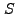= Expected total source counts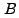= Expected background counts in the detect cell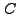= Total counts in a square detect cell of size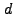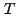= Total counts in the detect cell plus surrounding background frame (a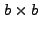box)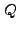=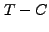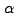= Integral of the point spread function (PSF) over the detect cell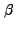= Integral of the PSF over the detect cell and surrounding background frame

There are three cases that need to be considered here:

1. Background is estimated from the background frame

2. Background is estimated from the background map or background value and background uncertainty is known

3. Background is estimated from the background map or background value and background uncertainty is negligible

We will consider these three cases in order.

## 6.2.1 Background Estimated from the Background Frame

We have: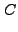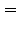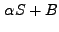(6.1)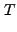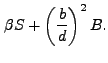(6.2)

Solving for, we get: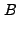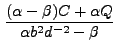(6.3)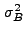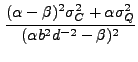(6.4)

Solving for, we get: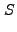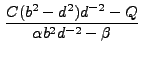(6.5)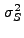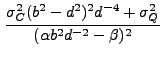(6.6)

S/N is then: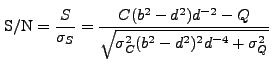(6.7)

Note that the estimate for S/N in Eq. (6.7) is independent ofand.

The Gehrels' (1986) formula for uncertainty is used: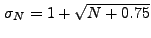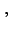(6.8)

where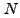can be eitheror. This simple formula gives excellent approximation for uncertainty (better than 1.5% for smalland better than a fraction of a percent for large). In this case, we have:(6.9)

## 6.2.2 Estimating Background with Known Uncertaintyand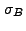are directly calculated from either the background value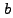: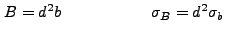or from the background map: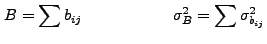where summing is done over the detect cell, and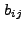and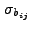are the values in the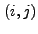pixel of the background map and background error map, respectively.

The equation for S/N becomes: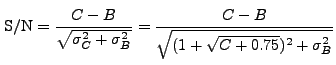(6.10)

## 6.2.3 Estimating Background with Negligible Uncertainty

The equation for source counts reduces to: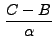(6.11)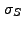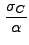(6.12)

S/N is equal to: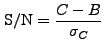(6.13)

or, using Eq. (6.8):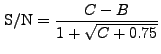(6.14)

# 6.3 Cell Size

The Chandra PSF varies strongly with the off-axis angle and photon energy. Therefore, different detect cell sizes are appropriate for different spatial regions and energies.

The user specifies the basis for the calculation of the cell size by providing the reference energy band and desired encircled energy to be encompassed in the detect cell. At each position, celldetect calculates the radius in pixels at which PSF reaches this encircled energy for a given combination of telescope, detector and grating, and then rounds the "diameter'' (i.e. radius times 2) up to the nearest multiple of 3 pixels. The rounding is done because the search for detections moves in steps of 1/3 of a detect cell.

Experience with the EINSTEIN and Rosat detection software indicates that the optimum cell size is approximately 3.6 times the sigma of the PSF (Harnden et al. 1984). For a Gaussian PSF, that corresponds to an encircled energy seting of 0.80.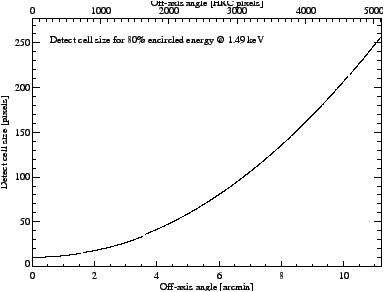Figure 6.1 shows growth of the cell size as a function of off-axis angle for the HRC-I detector.

# 6.4 Background Frame

If the local background option is used, the background counts are estimated from a frame surrounding the detect cell. The background frame size is selected to give the area of the background frame roughly twice the size of the detect cell. This makes the detect cell and the background "annulus'' similar in size. The parity of the background cell size is the same as of the detect cell size, so that the background frame and detect cell are centered on the same pixel and there are no asymmetries.

# 6.5 Output Options

The user provides the S/N threshold value (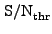). The tool moves across the data in steps of 1/3 of the local detect cell size searching for locations in which: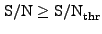(6.15)

The tool gives three options for detection output: all detections, local S/N peaks, and centroids of events in detect cells.

## 6.5.1 All Detections

celldetect can output all detect cells in which the condition from Eq. (6.15) is fulfilled. In this case, the output information should not be taken literally as a list of sources, since a single source will in all likelihood be reported several times. This option is, however, very useful if external background information is provided and the presence of extended sources is suspected, since it enables the user to identify regions in which there is an excess of events over the background.

## 6.5.2 Local S/N Peaks

celldetect can output detect cells which fulfill the condition from Eq. (6.15) and which are local S/N peaks, i.e. all their neighboring detect cells have lower S/N. Two cells are considered neighbors if the distance between their centers is not greater than 1/3 of the size of the smaller of the cells.

## 6.5.3 Centroid of Events in Detect Cells

This option is useful for isolated point sources, since the centroid gives a very accurate estimate of the position of the source. The centroids are calculated by finding the center of gravity for events in the detect cell: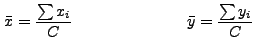(6.16)

where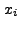,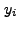are coordinates of events in the detect cell,is the total count of events in the cell, and summation is performed over the spatial extent of the cell.

In this case, the tool calculates centroid uncertainties, as well as the principal axes of the event distribution in the detect cell and the position angle of the major axis. These quantities are calculated utilizing the fact that the square of the principal axes of the distribution of events is mathematically equivalent to the principal moments of inertia for an ensemble of unit mass points.

Let us define: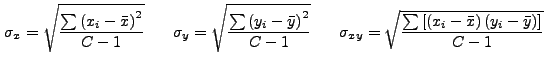(6.17)

Centroid uncertainties are: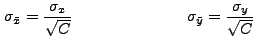(6.18)

The details of the procedure for calculating the principal axes and the position angle of the major axis can be found in any book on classical dynamics (e.g. Marion 1970). Here we will only list the final formulae for the major and minor axes -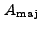and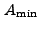, respectively - and the position angle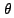of the major axis.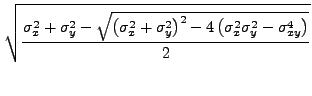(6.19)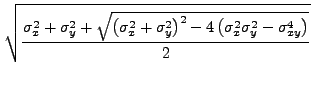(6.20)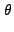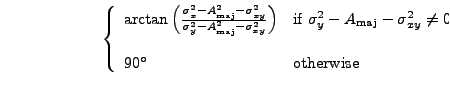(6.21)

# 6.6 References

Gehrels, N. 1996 ApJ, 303, 336

Harnden, F. R., Fabricant, D. G., Harris, D. E., & Schwartz, J. 1984, SAO Report No. 393

Marion, J. B. 1970, Classical Dynamics of Particles and Systems, Academic Press, New York